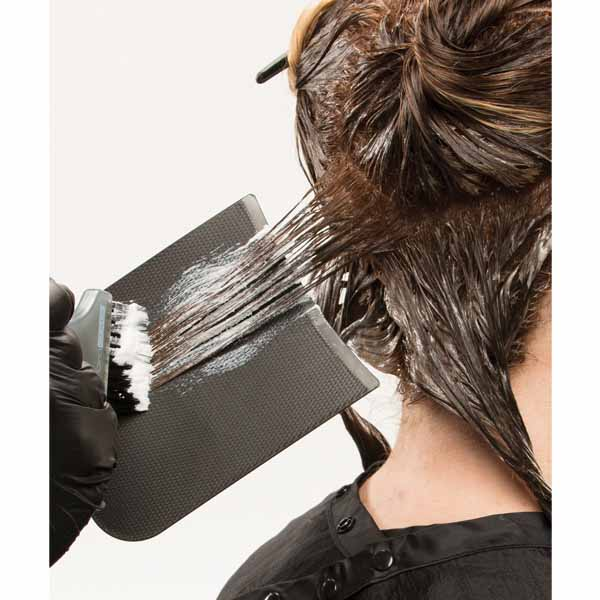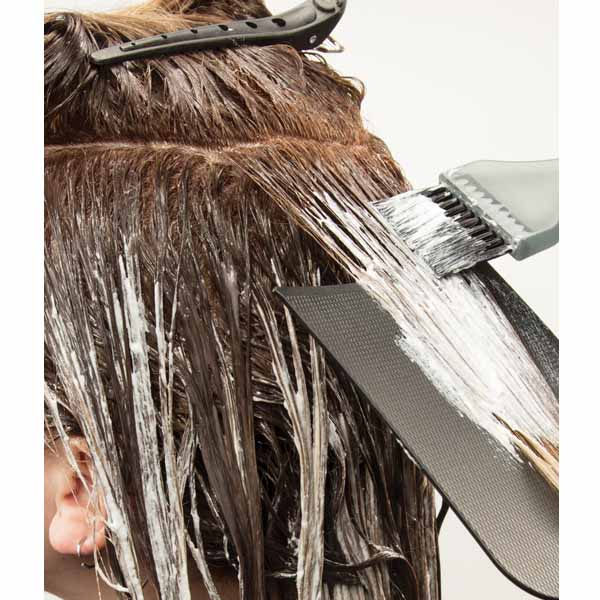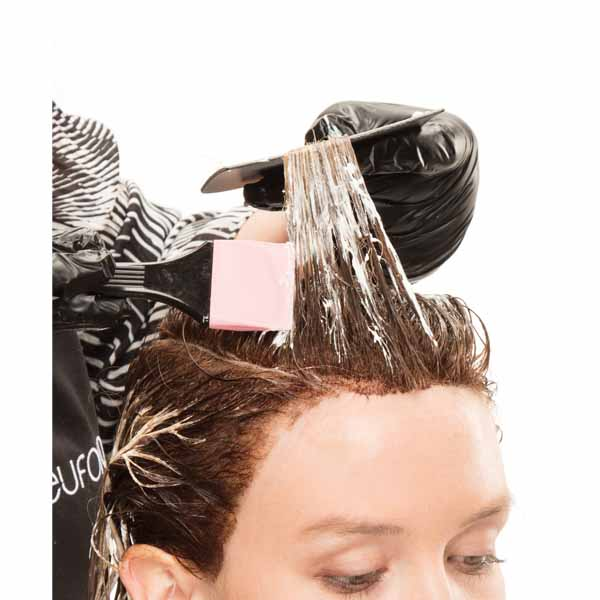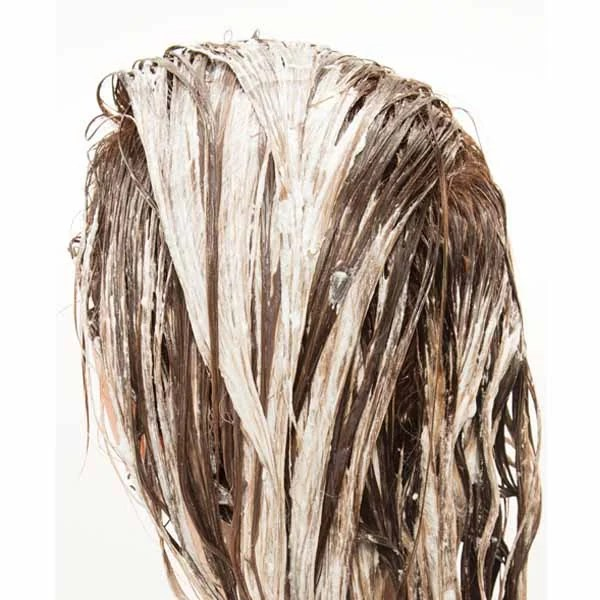Buttery Blonde
Last updated: June 30, 2017

# Buttery Blonde

ALL PHOTOS SLIDESHOW
GET THE HOW-TO• Formula A

Equal Parts 8.3/8G + 8.32/8GV + 10-Volume Developer (1:1.5)

• Formula B

Aloe Lite Cream Lightener + 30-Volume Developer (1:2)

• Formula C

Aloe Lite Cream Lightener + 15-Volume Developer (1:2)

• Formula D

Equal Parts 10.12/10AV + 10.32/10GV + 7-Volume Developer (1:2)

• Formula E

10.12/10AV + 7-Volume Developer (1:2)# Buttery Blonde

Écaille—translating to tortoise shell in English—merges classic beauty with a modern twist. This coloring technique from Eufora gives a fresh combination of balayage and base color to create a gorgeous effect, giving the look the translucent depth and tonality of its namesake. Get the steps to achieve this buttery blonde look!

Manufacturer: Eufora, @euforainternational

## COLOR FORMULAS• Formula A

Equal Parts 8.3/8G + 8.32/8GV + 10-Volume Developer (1:1.5)

• Formula B

Aloe Lite Cream Lightener + 30-Volume Developer (1:2)

• Formula C

Aloe Lite Cream Lightener + 15-Volume Developer (1:2)

• Formula D

Equal Parts 10.12/10AV + 10.32/10GV + 7-Volume Developer (1:2)

• Formula E

10.12/10AV + 7-Volume Developer (1:2)

## HOW-TO STEPS•• Formula A

Equal Parts 8.3/8G + 8.32/8GV + 10-Volume Developer (1:1.5)

• Formula B

Aloe Lite Cream Lightener + 30-Volume Developer (1:2)

• Formula C

Aloe Lite Cream Lightener + 15-Volume Developer (1:2)

• Formula D

Equal Parts 10.12/10AV + 10.32/10GV + 7-Volume Developer (1:2)

• Formula E

10.12/10AV + 7-Volume Developer (1:2)

1

Apply Formula A to the base.

•• Formula A

Equal Parts 8.3/8G + 8.32/8GV + 10-Volume Developer (1:1.5)

• Formula B

Aloe Lite Cream Lightener + 30-Volume Developer (1:2)

• Formula C

Aloe Lite Cream Lightener + 15-Volume Developer (1:2)

• Formula D

Equal Parts 10.12/10AV + 10.32/10GV + 7-Volume Developer (1:2)

• Formula E

10.12/10AV + 7-Volume Developer (1:2)

2

Section the hair.

•• Formula A

Equal Parts 8.3/8G + 8.32/8GV + 10-Volume Developer (1:1.5)

• Formula B

Aloe Lite Cream Lightener + 30-Volume Developer (1:2)

• Formula C

Aloe Lite Cream Lightener + 15-Volume Developer (1:2)

• Formula D

Equal Parts 10.12/10AV + 10.32/10GV + 7-Volume Developer (1:2)

• Formula E

10.12/10AV + 7-Volume Developer (1:2)

3

Pull Formula A through Zone 1.

•• Formula A

Equal Parts 8.3/8G + 8.32/8GV + 10-Volume Developer (1:1.5)

• Formula B

Aloe Lite Cream Lightener + 30-Volume Developer (1:2)

• Formula C

Aloe Lite Cream Lightener + 15-Volume Developer (1:2)

• Formula D

Equal Parts 10.12/10AV + 10.32/10GV + 7-Volume Developer (1:2)

• Formula E

10.12/10AV + 7-Volume Developer (1:2)

4

Starting in the center back of Zone 2, apply Formula A in ¼ inch alternating diagonal back sections. Using a balayage board, apply Formula B to the mid-section of the hair and Formula C to the ends.

•• Formula A

Equal Parts 8.3/8G + 8.32/8GV + 10-Volume Developer (1:1.5)

• Formula B

Aloe Lite Cream Lightener + 30-Volume Developer (1:2)

• Formula C

Aloe Lite Cream Lightener + 15-Volume Developer (1:2)

• Formula D

Equal Parts 10.12/10AV + 10.32/10GV + 7-Volume Developer (1:2)

• Formula E

10.12/10AV + 7-Volume Developer (1:2)

5

Apply Formula A to the midshaft through the ends. Repeat alternating Steps 4 and 5 until you reach the hairline, finishing with a light section at the side hairline.

•• Formula A

Equal Parts 8.3/8G + 8.32/8GV + 10-Volume Developer (1:1.5)

• Formula B

Aloe Lite Cream Lightener + 30-Volume Developer (1:2)

• Formula C

Aloe Lite Cream Lightener + 15-Volume Developer (1:2)

• Formula D

Equal Parts 10.12/10AV + 10.32/10GV + 7-Volume Developer (1:2)

• Formula E

10.12/10AV + 7-Volume Developer (1:2)

6

Starting in the back of Zone 1, work in ¼ inch subsections from the back natural part to the front hairline.

•• Formula A

Equal Parts 8.3/8G + 8.32/8GV + 10-Volume Developer (1:1.5)

• Formula B

Aloe Lite Cream Lightener + 30-Volume Developer (1:2)

• Formula C

Aloe Lite Cream Lightener + 15-Volume Developer (1:2)

• Formula D

Equal Parts 10.12/10AV + 10.32/10GV + 7-Volume Developer (1:2)

• Formula E

10.12/10AV + 7-Volume Developer (1:2)

7

Repeat color formulas and techniques from Steps 4 and 5.

•• Formula A

Equal Parts 8.3/8G + 8.32/8GV + 10-Volume Developer (1:1.5)

• Formula B

Aloe Lite Cream Lightener + 30-Volume Developer (1:2)

• Formula C

Aloe Lite Cream Lightener + 15-Volume Developer (1:2)

• Formula D

Equal Parts 10.12/10AV + 10.32/10GV + 7-Volume Developer (1:2)

• Formula E

10.12/10AV + 7-Volume Developer (1:2)

8

Allow hair to process, rinse thoroughly and gently wash with Beautifying Elixirs Shampoo. Do not condition. Tone the mid-section with Formula D and the ends with Formula E. Process for 20 minutes and rinse thoroughly. Apply Eufora Color Elixir™ Professional Sealer and rinse.

•• Formula A

Equal Parts 8.3/8G + 8.32/8GV + 10-Volume Developer (1:1.5)

• Formula B

Aloe Lite Cream Lightener + 30-Volume Developer (1:2)

• Formula C

Aloe Lite Cream Lightener + 15-Volume Developer (1:2)

• Formula D

Equal Parts 10.12/10AV + 10.32/10GV + 7-Volume Developer (1:2)

• Formula E

10.12/10AV + 7-Volume Developer (1:2)

9

Finished Look